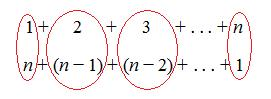# Clever Carl

##### Age 7 to 14

Published 1999 Revised 2012Carl Friedrich Gauss (1777-1855) is recognised as being one of the greatest mathematicians of all time. During his lifetime he made significant contributions to almost every area of mathematics, as well as physics, astronomy and statistics. Like many of the great mathematicians, Gauss showed amazing mathematical skill from an early age, and there are many stories which show how clever he could be.

The most well-known story is a tale from when Gauss was still at primary school. One day Gauss' teacher asked his class to add together all the numbers from $1$ to $100$, assuming that this task would occupy them for quite a while. He was shocked when young Gauss, after a few seconds thought, wrote down the answer $5050$. The teacher couldn't understand how his pupil had calculated the sum so quickly in his head, but the eight year old Gauss pointed out that the problem was actually quite simple.

He had added the numbers in pairs - the first and the last, the second and the second to last and so on, observing that $1+100=101$, $2+99=101$, $3+98=101$, ...so the total would be $50$ lots of $101$, which is $5050$.

It is remarkable that a child still in elementary school had discovered this method for summing sequences of numbers, but of course Gauss was a remarkable child. Fortunately his talents were discovered, and he was given the chance to study at university. By his early twenties, Gauss had made discoveries that would shape the future of mathematics.

While the story may not be entirely true, it is a popular tale for maths teachers to tell because it shows that Gauss had a natural insight into mathematics. Rather than performing a great feat of mental arithmetic, Gauss had seen the structure of the problem and used it to find a short cut to a solution.

Gauss could have used his method to add all the numbers from $1$ to any number - by pairing off the first number with the last, the second number with the second to last, and so on, he only had to multiply this total by half the last number, just one swift calculation.

Can you see how Gauss's method works? Try using it to work out the total of all the numbers from $1$ to $10$. What about $1$ to $50$? The answers are at the bottom of this page.

Or why not challenge a friend to add up the numbers from $1$ to a nice large number, and then amaze them by getting the answer in seconds!

The rest of the article explains how you could use algebra to write Gauss's method - if you haven't yet learned any algebra you may wish to skip this part.

Gauss's method forms a general formula for the sum of the first $n$ integers, namely that $$1+2+3+\ldots +n=\frac{1}{2}n(n+1)$$
One way of presenting Gauss' method is to write out the sum twice, the second time reversing it as shown.If we add both rows we get the sum of $1$ to $n$, but twice. Gauss added the rows pairwise - each pair adds up to $n+1$ and there are $n$ pairs, so the sum of the rows is also $n\times (n+1)$. It follows that $2\times (1+2+\ldots +n) = n\times (n+1)$, from which we obtain the formula.
Gauss' formula is a result of counting a quantity in a clever way. The problems Picturing Triangular Numbers, Mystic Rose, and Handshakes all use similar clever counting to come up with a formula for adding numbers.

Answers: total from 1 to 10 = 55, total from 1 to 50 = 1275